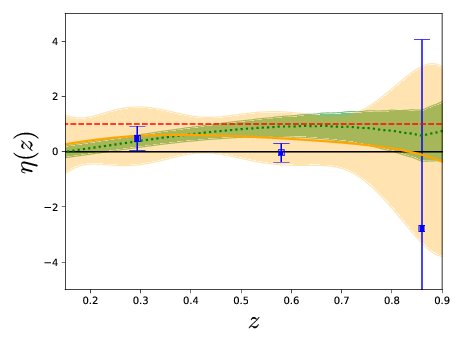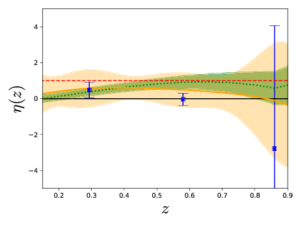# Model-independent reconstruction of the linear anisotropic stressAuthors: Ana Marta Pinho,  Santiago Casas, Luca Amendola Journal: Accepted for JCAP Year: 05/2018 Download: Inspire| Arxiv

## Abstract

In this work, we use recent data on the Hubble expansion rate H(z), the quantity fσ8(z) from redshift space distortions and the statistic Eg from clustering and lensing observables to constrain in a model-independent way the linear anisotropic stress parameter η. This estimate is free of assumptions about initial conditions, bias, the abundance of dark matter and the background expansion. We denote this observable estimator as ηobs. If ηobs turns out to be different from unity, it would imply either a modification of gravity or a non-perfect fluid form of dark energy clustering at sub-horizon scales. Using three different methods to reconstruct the underlying model from data, we report the value of ηobs at three redshift values, z=0.29,0.58,0.86. Using the method of polynomial regression, we find ηobs=0.57±1.05, ηobs=0.48±0.96, and ηobs=0.11±3.21, respectively. Assuming a constant ηobs in this range, we find ηobs=0.49±0.69. We consider this method as our fiducial result, for reasons clarified in the text. The other two methods give for a constant anisotropic stress ηobs=0.15±0.27 (binning) and ηobs=0.53±0.19 (Gaussian Process). We find that all three estimates are compatible with each other within their 1σ error bars. While the polynomial regression method is compatible with standard gravity, the other two methods are in tension with it.